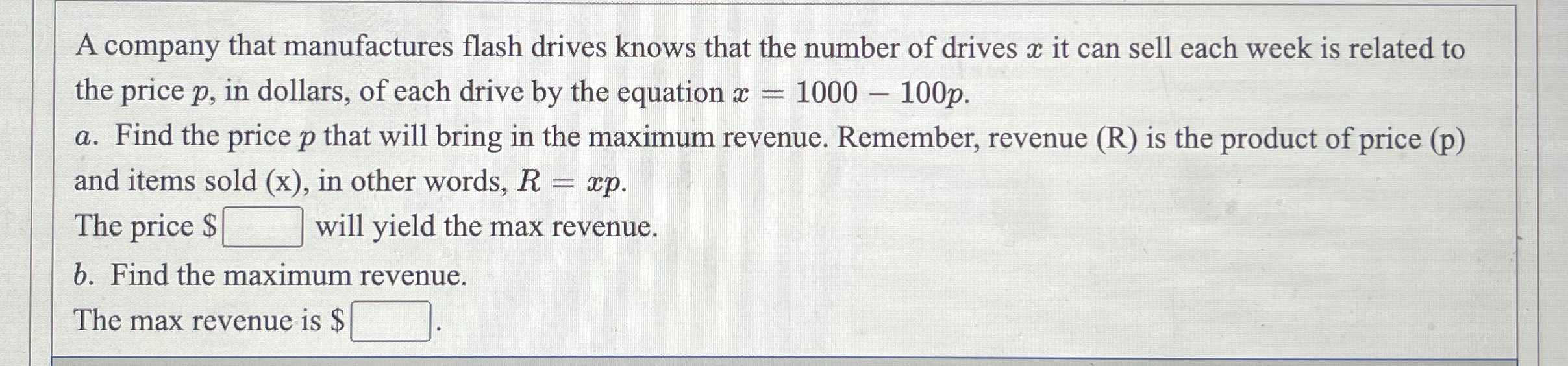### ¿Todavía tienes preguntas de matemáticas?

Pregunte a nuestros tutores expertos
Algebra
PreguntaA company that manufactures flash drives knows that the number of drives $$x$$ it can sell each week is related to the price $$p$$ , in dollars, of each drive by the equation $$x = 1000 - 100 p$$ .

a. Find the price $$p$$ that will bring in the maximum revenue. Remember, revenue $$( R )$$ is the product of price (p) and items sold $$( x )$$ , in other words, $$R = x p$$ . The price $$\ \square$$ will yield the max revenue.

b. Find the maximum revenue. The max revenue is $$\ \square .$$